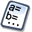Logiciel libre de géométrie, d'analyse et de simulation multiplateforme par Yves Biton

# 7.4.4 version of MathGraph32 : Improvements on matrices and ergonomy

modification samedi 25 février 2023.

Toutes les versions de cet article : [English] [français]

7.4.4 version of MathGraph32 brings up the following improvements :

Creation of matrices :

• Creating a matrix by a formula for each cell, the number of cells is now limited to 200.
• Creating a matrix with random integer values, the number of cells is now limites to 100 000.

Ergonomy improvements :

• Using the tool of numerical object modification (icon), when an object was reclassified of deleted, the first object of the list was selected. Now, if you reclassify an object, this object remains selected and, when yo delete an object, the nearest objet is selected after the deletion.

7.4.4 version fixes up the following bug :

• Si A et B were matrices and, for instance, a real calculation named x was created with the formula A(1,B(1,2)) eand the matrix B was then deleted, calculation x was not deleted which produced a non viable figure (once saved on a file il could’t be reopened).

7.4.4 fixes up the following flaw :

• When implementing a macro construction, it could happen that some dynamic LaTeX display would not be correctly updated.